### Home > CALC > Chapter 5 > Lesson 5.5.2 > Problem5-169

5-169.
1. Find the antiderivative of each function. Homework Help ✎

1. f(x) = sin x

2.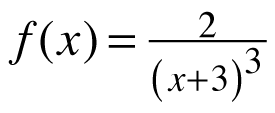3. f(x) = sec2 x

4.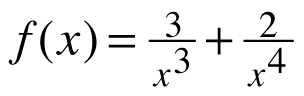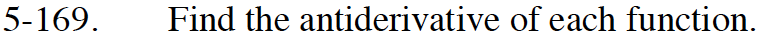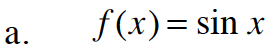Don't forget the + C.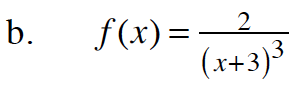Think of this as f(x) = 2(x + 3)–3.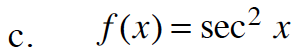What common trig function has a derivative of y = sec²x?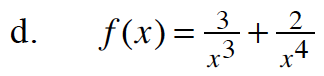Before you find F(x), rewrite f(x) with a negative exponent.